# Approximating the Riemann-Stieltjes integral of smooth integrands and of bounded variation integrators

## Abstract

In the present paper, we investigate the problem of approximating the Riemann-Stieltjes integral ${\int }_{a}^{b}f\left(\lambda \right)\phantom{\rule{0.2em}{0ex}}du\left(\lambda \right)$ in the case when the integrand f is n-time differentiable and the derivative ${f}^{\left(n\right)}$ is either of locally bounded variation, or Lipschitzian on an interval incorporating $\left[a,b\right]$. A priory error bounds for several classes of integrators u and applications in approximating the finite Laplace-Stieltjes transform and the finite Fourier-Stieltjes sine and cosine transforms are provided as well.

MSC:41A51, 26D15, 26D10.

## 1 Introduction

The concept of Riemann-Stieltjes integral ${\int }_{a}^{b}f\left(t\right)\phantom{\rule{0.2em}{0ex}}du\left(t\right)$, where f is called the integrand, u is called the integrator, plays an important role in mathematics, for instance in the definition of complex integral, the representation of bounded linear functionals on the Banach space of all continuous functions on an interval $\left[a,b\right]$, in the spectral representation of selfadjoint operators on complex Hilbert spaces and other classes of operators such as the unitary operators, etc.

However, the numerical analysis of this integral is quite poor as pointed out by the seminal paper due to Michael Tortorella from 1990 . Earlier results in this direction, however, were provided by Dubuc and Todor in their 1984 and 1987 papers [2, 3] and , respectively. For recent results concerning the approximation of the Riemann-Stieltjes integral, see the work of Diethelm , Liu , Mercer , Munteanu , Mozyrska et al.  and the references therein. For other recent results obtained in the same direction by the first author and his colleagues from RGMIA, see  and . A comprehensive list of preprints related to this subject may be found at http://rgmia.org.

In order to approximate the Riemann-Stieltjes integral ${\int }_{a}^{b}p\left(t\right)\phantom{\rule{0.2em}{0ex}}dv\left(t\right)$, where $p,v:\left[a,b\right]\to \mathbb{R}$ are functions for which the above integral exists, Dragomir established in  the following integral identity: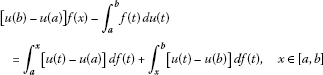(1.1)

provided that the involved integrals exist. In the particular case when $u\left(t\right)=t$, $t\in \left[a,b\right]$, the above identity reduces to the celebrated Montgomery identity (see [, p.565]) that has been extensively used by many authors in obtaining various inequalities of Ostrowski type. For a comprehensive recent collection of works related to Ostrowski’s inequality, see the book , the papers [1012, 2132] and . For other results concerning error bounds of quadrature rules related to midpoint and trapezoid rules, see  and the references therein.

Motivated by the recent results from [18, 46, 47] (see also [11, 27] and ) in the present paper we investigate the problem of approximating the Riemann-Stieltjes integral ${\int }_{a}^{b}f\left(\lambda \right)\phantom{\rule{0.2em}{0ex}}du\left(\lambda \right)$ in the case when the integrand f is n-times differentiable and the derivative ${f}^{\left(n\right)}$ is either of locally bounded variation, or Lipschitzian on an interval incorporating $\left[a,b\right]$. A priori error bounds for several classes of integrators u and applications in approximating the finite Laplace-Stieltjes transform and the finite Fourier-Stieltjes sine and cosine transforms are provided as well.

## 2 Some representation results

In this section, we establish some representation results for the Riemann-Stieltjes integral when the integrand is n-times differentiable and the integrator is of locally bounded variation. Several particular cases of interest are considered as well.

Theorem 1 Assume that the function $f:I\to \mathbb{C}$ is n-times differentiable on the interiorof the interval I ($n\ge 1$) and the nth derivative ${f}^{\left(n\right)}$ is of locally bounded variation on. If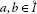with $a, $c\in \left[a,b\right]$ and $u:\left[a,b\right]\to \mathbb{C}$ is of bounded variation on $\left[a,b\right]$, then the Riemann-Stieltjes integral ${\int }_{a}^{b}f\left(\lambda \right)\phantom{\rule{0.2em}{0ex}}du\left(\lambda \right)$ exists, we have the identity

${\int }_{a}^{b}f\left(\lambda \right)\phantom{\rule{0.2em}{0ex}}du\left(\lambda \right)={T}_{n}\left(f,u,a,c,b\right)+{R}_{n}\left(f,u,a,c,b\right),$
(2.1)

where

$\begin{array}{rcl}{T}_{n}\left(f,u,a,c,b\right)& :=& \sum _{k=0}^{n}\frac{1}{k!}{f}^{\left(k\right)}\left(c\right)\left[{\left(b-c\right)}^{k}u\left(b\right)+{\left(-1\right)}^{k+1}{\left(c-a\right)}^{k}u\left(a\right)\right]\\ -\sum _{k=0}^{n-1}\frac{1}{k!}{f}^{\left(k+1\right)}\left(c\right){\int }_{a}^{b}{\left(\lambda -c\right)}^{k}u\left(\lambda \right)\phantom{\rule{0.2em}{0ex}}d\lambda \end{array}$
(2.2)

and the remainder ${R}_{n}\left(f,u,a,c,b\right)$ can be represented as

${R}_{n}\left(f,u,a,c,b\right):=\frac{1}{n!}{\int }_{a}^{b}\left({\int }_{c}^{\lambda }{\left(\lambda -t\right)}^{n}\phantom{\rule{0.2em}{0ex}}d{f}^{\left(n\right)}\left(t\right)\right)\phantom{\rule{0.2em}{0ex}}du\left(\lambda \right).$
(2.3)

Both integrals in (2.3) are taken in the Riemann-Stieltjes sense.

Proof

Under the assumption of the theorem, we utilize the following Taylor’s representation

$f\left(\lambda \right)=\sum _{k=0}^{n}\frac{1}{k!}{f}^{\left(k\right)}\left(c\right){\left(\lambda -c\right)}^{k}+\frac{1}{n!}{\int }_{c}^{\lambda }{\left(\lambda -t\right)}^{n}\phantom{\rule{0.2em}{0ex}}d{f}^{\left(n\right)}\left(t\right)$
(2.4)

that holds for any $c\in \left[a,b\right]$ and $n\ge 0$. The integral in (2.4) is taken in the Riemann-Stieltjes sense.

We can prove this equality by induction.

Indeed, for $n=0$, we have

$f\left(\lambda \right)=f\left(c\right)+{\int }_{c}^{\lambda }\phantom{\rule{0.2em}{0ex}}df\left(t\right)$

that holds for any function of locally bounded variation on.

Now, assume that (2.4) is true for an $n\ge 0$ and let us prove that it holds for ‘$n+1$’, namely

$f\left(\lambda \right)=\sum _{k=0}^{n+1}\frac{1}{k!}{f}^{\left(k\right)}\left(c\right){\left(\lambda -c\right)}^{k}+\frac{1}{\left(n+1\right)!}{\int }_{c}^{\lambda }{\left(\lambda -t\right)}^{n+1}\phantom{\rule{0.2em}{0ex}}d{f}^{\left(n+1\right)}\left(t\right)$
(2.5)

provided that the function $f:I\to \mathbb{C}$ is $\left(n+1\right)$-times differentiable on the interiorof the interval I and the $\left(n+1\right)$-th derivative ${f}^{\left(n+1\right)}$ is of locally bounded variation on.

Utilizing the integration by parts formula for the Riemann-Stieltjes integral and the reduction of the Riemann-Stieltjes integral to a Riemann integral (see, for instance, ) we have: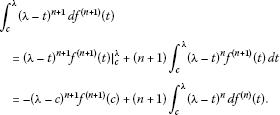(2.6)

From (2.4), we have that

${\int }_{c}^{\lambda }{\left(\lambda -t\right)}^{n}\phantom{\rule{0.2em}{0ex}}d{f}^{\left(n\right)}\left(t\right)=\left[f\left(\lambda \right)-\sum _{k=0}^{n}\frac{1}{k!}{f}^{\left(k\right)}\left(c\right){\left(\lambda -c\right)}^{k}\right]n!$

which inserted in the last part of (2.6) provides the equality

$\begin{array}{rcl}{\int }_{c}^{\lambda }{\left(\lambda -t\right)}^{n+1}\phantom{\rule{0.2em}{0ex}}d{f}^{\left(n+1\right)}\left(t\right)& =& -{\left(\lambda -c\right)}^{n+1}{f}^{\left(n+1\right)}\left(c\right)\\ +\left(n+1\right)!\left[f\left(\lambda \right)-\sum _{k=0}^{n}\frac{1}{k!}{f}^{\left(k\right)}\left(c\right){\left(\lambda -c\right)}^{k}\right].\end{array}$
(2.7)

We observe that, by division with $\left(n+1\right)!$, the equality (2.7) becomes the desired representation (2.5).

Further on, from the identity (2.4) we obtain

$\begin{array}{rcl}{\int }_{a}^{b}f\left(\lambda \right)\phantom{\rule{0.2em}{0ex}}du\left(\lambda \right)& =& \sum _{k=0}^{n}\frac{1}{k!}{f}^{\left(k\right)}\left(c\right){\int }_{a}^{b}{\left(\lambda -c\right)}^{k}\phantom{\rule{0.2em}{0ex}}du\left(\lambda \right)\\ +\frac{1}{n!}{\int }_{a}^{b}\left({\int }_{c}^{\lambda }{\left(\lambda -t\right)}^{n}\phantom{\rule{0.2em}{0ex}}d{f}^{\left(n\right)}\left(t\right)\right)\phantom{\rule{0.2em}{0ex}}du\left(\lambda \right).\end{array}$
(2.8)

Utilizing the integration by parts formula, we have for $k\ge 1$ that

$\begin{array}{rcl}{\int }_{a}^{b}{\left(\lambda -c\right)}^{k}\phantom{\rule{0.2em}{0ex}}du\left(\lambda \right)& =& {\left(\lambda -c\right)}^{k}u\left(\lambda \right){|}_{a}^{b}-k{\int }_{a}^{b}{\left(\lambda -c\right)}^{k-1}u\left(\lambda \right)\phantom{\rule{0.2em}{0ex}}d\lambda \\ =& {\left(b-c\right)}^{k}u\left(b\right)+{\left(-1\right)}^{k+1}{\left(c-a\right)}^{k}u\left(a\right)-k{\int }_{a}^{b}{\left(\lambda -c\right)}^{k-1}u\left(\lambda \right)\phantom{\rule{0.2em}{0ex}}d\lambda .\end{array}$
(2.9)

For $k=0$, we have ${\int }_{a}^{b}\phantom{\rule{0.2em}{0ex}}du\left(\lambda \right)=u\left(b\right)-u\left(a\right)$.

Therefore, by (2.9) we get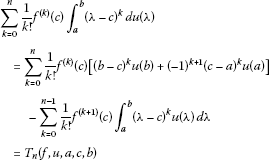(2.10)

and by (2.8) the representation (2.1) is thus obtained.

This completes the proof. □

Remark 1 Assume that the function $f:I\to \mathbb{C}$ is n-times differentiable on the interiorof the interval I ($n\ge 1$) and the n th derivative ${f}^{\left(n\right)}$ is of locally bounded variation on. Ifwith $a and $u:\left[a,b\right]\to \mathbb{C}$ is of bounded variation on $\left[a,b\right]$, then, by choosing $c=a$ in the formulae above we have

$\begin{array}{rcl}{}_{d}D_{n}\left(f,u,a,b\right)& :=& {T}_{n}\left(f,u,a,a,b\right)\\ =& \sum _{k=0}^{n}\frac{1}{k!}{f}^{\left(k\right)}\left(a\right){\left(b-a\right)}^{k}u\left(b\right)-\sum _{k=0}^{n-1}\frac{1}{k!}{f}^{\left(k+1\right)}\left(a\right){\int }_{a}^{b}{\left(\lambda -a\right)}^{k}u\left(\lambda \right)\phantom{\rule{0.2em}{0ex}}d\lambda \end{array}$
(2.11)

and

${}_{d}R_{n}\left(f,u,a,b\right):={R}_{n}\left(f,u,a,a,b\right)=\frac{1}{n!}{\int }_{a}^{b}\left({\int }_{a}^{\lambda }{\left(\lambda -t\right)}^{n}\phantom{\rule{0.2em}{0ex}}d{f}^{\left(n\right)}\left(t\right)\right)\phantom{\rule{0.2em}{0ex}}du\left(\lambda \right).$
(2.12)

This give the representation

${\int }_{a}^{b}f\left(\lambda \right)\phantom{\rule{0.2em}{0ex}}du\left(\lambda \right){=}_{d}{D}_{n}\left(f,u,a,b\right){+}_{d}{R}_{n}\left(f,u,a,b\right).$
(2.13)

Now, if we choose $c=\frac{a+b}{2}$, then we have

$\begin{array}{rcl}{M}_{n}\left(f,u,a,b\right)& :=& {T}_{n}\left(f,u,a,\frac{a+b}{2},b\right)\\ =& \sum _{k=0}^{n}\frac{1}{k!{2}^{k}}{f}^{\left(k\right)}\left(\frac{a+b}{2}\right){\left(b-a\right)}^{k}\left[u\left(b\right)+{\left(-1\right)}^{k+1}u\left(a\right)\right]\\ -\sum _{k=0}^{n-1}\frac{1}{k!}{f}^{\left(k+1\right)}\left(\frac{a+b}{2}\right){\int }_{a}^{b}{\left(\lambda -\frac{a+b}{2}\right)}^{k}u\left(\lambda \right)\phantom{\rule{0.2em}{0ex}}d\lambda \end{array}$
(2.14)

and

$\begin{array}{rcl}{}_{M}R_{n}\left(f,u,a,b\right)& :=& {R}_{n}\left(f,u,a,\frac{a+b}{2},b\right)\\ =& \frac{1}{n!}{\int }_{a}^{b}\left({\int }_{\frac{a+b}{2}}^{\lambda }{\left(\lambda -t\right)}^{n}\phantom{\rule{0.2em}{0ex}}d{f}^{\left(n\right)}\left(t\right)\right)\phantom{\rule{0.2em}{0ex}}du\left(\lambda \right),\end{array}$
(2.15)

which provide the representation

${\int }_{a}^{b}f\left(\lambda \right)\phantom{\rule{0.2em}{0ex}}du\left(\lambda \right)={M}_{n}\left(f,u,a,b\right){+}_{M}{R}_{n}\left(f,u,a,b\right).$
(2.16)

Finally, if we choose $c=b$, then we have

$\begin{array}{rcl}{}_{u}D_{n}\left(f,u,a,b\right)& :=& {T}_{n}\left(f,u,a,b,b\right)\\ =& \sum _{k=0}^{n}\frac{1}{k!}{f}^{\left(k\right)}\left(b\right){\left(-1\right)}^{k+1}{\left(b-a\right)}^{k}u\left(a\right)\\ +\sum _{k=0}^{n-1}\frac{{\left(-1\right)}^{k+1}}{k!}{f}^{\left(k+1\right)}\left(b\right){\int }_{a}^{b}{\left(b-\lambda \right)}^{k}u\left(\lambda \right)\phantom{\rule{0.2em}{0ex}}d\lambda \end{array}$
(2.17)

and the remainder

$\begin{array}{rcl}{}_{u}R_{n}\left(f,u,a,b\right)& :=& {R}_{n}\left(f,u,a,b,b\right)\\ =& \frac{{\left(-1\right)}^{n+1}}{n!}{\int }_{a}^{b}\left({\int }_{\lambda }^{b}{\left(t-\lambda \right)}^{n}\phantom{\rule{0.2em}{0ex}}d{f}^{\left(n\right)}\left(t\right)\right)\phantom{\rule{0.2em}{0ex}}du\left(\lambda \right).\end{array}$
(2.18)

Making use of (2.1) we get

${\int }_{a}^{b}f\left(\lambda \right)\phantom{\rule{0.2em}{0ex}}du\left(\lambda \right){=}_{u}{D}_{n}\left(f,u,a,b\right){+}_{u}{R}_{n}\left(f,u,a,b\right).$
(2.19)

## 3 Error bounds

In order to provide sharp error bounds in the approximation rules outlined above, we need the following well-known lemma concerning sharp estimates for the Riemann-Stieltjes integral for various pairs of integrands and integrators (see, for instance, ).

Lemma 1 Let $p,v:\left[a,b\right]\to \mathbb{C}$ two bounded functions on the compact interval $\left[a,b\right]$.

1. (i)

If p is continuous and v is of bounded variation, then the Riemann-Stieltjes integral ${\int }_{a}^{b}p\left(t\right)\phantom{\rule{0.2em}{0ex}}dv\left(t\right)$ exists and

$|{\int }_{a}^{b}p\left(t\right)\phantom{\rule{0.2em}{0ex}}dv\left(t\right)|\le \underset{t\in \left[a,b\right]}{max}|p\left(t\right)|\underset{a}{\overset{b}{\bigvee }}\left(v\right),$
(3.1)

where ${\bigvee }_{a}^{b}\left(v\right)$ denotes the total variation of v on the interval $\left[a,b\right]$.

1. (ii)

If p is Riemann integrable and v is Lipschitzian with the constant $L>0$, i.e.,

$|v\left(t\right)-v\left(s\right)|\le L|t-s|\phantom{\rule{1em}{0ex}}\mathit{\text{for each}}\phantom{\rule{0.1em}{0ex}}t,s\in \left[a,b\right],$

then the Riemann-Stieltjes integral ${\int }_{a}^{b}p\left(t\right)\phantom{\rule{0.2em}{0ex}}dv\left(t\right)$ exists and

$|{\int }_{a}^{b}p\left(t\right)\phantom{\rule{0.2em}{0ex}}dv\left(t\right)|\le L{\int }_{a}^{b}|p\left(t\right)|\phantom{\rule{0.2em}{0ex}}dt\left(\le L\underset{t\in \left[a,b\right]}{sup}|p\left(t\right)|\left(b-a\right)\right).$
(3.2)

All the above inequalities are sharp in the sense that there are examples of functions for which each equality case is realized.

Utilizing this result concerning bounds for the Riemann-Stieltjes integral, we can provide the following error bounds in approximating the integral ${\int }_{a}^{b}f\left(\lambda \right)\phantom{\rule{0.2em}{0ex}}du\left(\lambda \right)$.

Theorem 2 Assume that the function $f:I\to \mathbb{C}$ is n-times differentiable on the interiorof the interval I ($n\ge 1$) and the nth derivative ${f}^{\left(n\right)}$ is of locally bounded variation on. Ifwith $a, $c\in \left[a,b\right]$ and $u:\left[a,b\right]\to \mathbb{C}$ is of bounded variation on $\left[a,b\right]$, then we have the representation (2.1), where the approximation term ${T}_{n}\left(f,u,a,c,b\right)$ is given by (2.2) and the remainder ${R}_{n}\left(f,u,a,c,b\right)$ satisfies the inequality

$|{R}_{n}\left(f,u,a,c,b\right)|\le \frac{1}{n!}{\left[\frac{1}{2}\left(b-a\right)+|c-\frac{a+b}{2}|\right]}^{n}\underset{a}{\overset{b}{\bigvee }}\left({f}^{\left(n\right)}\right)\underset{a}{\overset{b}{\bigvee }}\left(u\right),$
(3.3)

for any $c\in \left[a,b\right]$.

If the nth derivative ${f}^{\left(n\right)}$ is Lipschitzian with the constant ${L}_{n}>0$ on $\left[a,b\right]$, then we have

$|{R}_{n}\left(f,u,a,c,b\right)|\le \frac{1}{\left(n+1\right)!}{L}_{n}{\left[\frac{1}{2}\left(b-a\right)+|c-\frac{a+b}{2}|\right]}^{n+1}\underset{a}{\overset{b}{\bigvee }}\left(u\right),$
(3.4)

for any $c\in \left[a,b\right]$.

Proof

Utilizing the property (i) from Lemma 1, we have successively

$\begin{array}{rcl}|{R}_{n}\left(f,u,a,c,b\right)|& =& \frac{1}{n!}|{\int }_{a}^{b}\left({\int }_{c}^{\lambda }{\left(\lambda -t\right)}^{n}\phantom{\rule{0.2em}{0ex}}d{f}^{\left(n\right)}\left(t\right)\right)\phantom{\rule{0.2em}{0ex}}du\left(\lambda \right)|\\ \le & \frac{1}{n!}\underset{\lambda \in \left[a,b\right]}{max}|{\int }_{c}^{\lambda }{\left(\lambda -t\right)}^{n}\phantom{\rule{0.2em}{0ex}}d{f}^{\left(n\right)}\left(t\right)|\underset{a}{\overset{b}{\bigvee }}\left(u\right)\end{array}$
(3.5)

for any $c\in \left[a,b\right]$.

For $c,\lambda \in \left[a,b\right]$, denote

$B\left(\lambda ,c\right):=|{\int }_{c}^{\lambda }{\left(\lambda -t\right)}^{n}\phantom{\rule{0.2em}{0ex}}d{f}^{\left(n\right)}\left(t\right)|.$
(3.6)

By the property (i) from Lemma 1 applied for ${f}^{\left(n\right)}$ we have for $c<\lambda$ that

$\begin{array}{rcl}B\left(\lambda ,c\right)& \le & \underset{t\in \left[c,\lambda \right]}{max}|\lambda -t{|}^{n}\underset{c}{\overset{\lambda }{\bigvee }}\left({f}^{\left(n\right)}\right)\\ =& {\left(\lambda -c\right)}^{n}\underset{c}{\overset{\lambda }{\bigvee }}\left({f}^{\left(n\right)}\right)\le {\left(\lambda -c\right)}^{n}\underset{a}{\overset{b}{\bigvee }}\left({f}^{\left(n\right)}\right)\\ \le & {\left(b-c\right)}^{n}\underset{a}{\overset{b}{\bigvee }}\left({f}^{\left(n\right)}\right)\end{array}$

and for $c>\lambda$ that

$\begin{array}{rcl}B\left(\lambda ,c\right)& \le & \underset{t\in \left[\lambda ,c\right]}{max}|\lambda -t{|}^{n}\underset{\lambda }{\overset{c}{\bigvee }}\left({f}^{\left(n\right)}\right)\\ =& {\left(c-\lambda \right)}^{n}\underset{\lambda }{\overset{c}{\bigvee }}\left({f}^{\left(n\right)}\right)\le {\left(c-\lambda \right)}^{n}\underset{a}{\overset{b}{\bigvee }}\left({f}^{\left(n\right)}\right)\\ \le & {\left(c-a\right)}^{n}\underset{a}{\overset{b}{\bigvee }}\left({f}^{\left(n\right)}\right).\end{array}$

Therefore,

$\begin{array}{rcl}\underset{\lambda \in \left[a,b\right]}{max}B\left(\lambda ,c\right)& \le & max\left\{{\left(b-c\right)}^{n},{\left(c-a\right)}^{n}\right\}\underset{a}{\overset{b}{\bigvee }}\left({f}^{\left(n\right)}\right)\\ =& {\left[max\left\{b-c,c-a\right\}\right]}^{n}\underset{a}{\overset{b}{\bigvee }}\left({f}^{\left(n\right)}\right)\\ =& {\left[\frac{1}{2}\left(b-a\right)+|c-\frac{a+b}{2}|\right]}^{n}\underset{a}{\overset{b}{\bigvee }}\left({f}^{\left(n\right)}\right),\end{array}$
(3.7)

for any $c\in \left[a,b\right]$.

Utilizing (3.5) and (3.7), we deduce the desired inequality (3.3).

By the property (ii) from Lemma 1 applied for ${f}^{\left(n\right)}$, we have that

$B\left(\lambda ,c\right)\le {L}_{n}|{\int }_{c}^{\lambda }|\lambda -t{|}^{n}\phantom{\rule{0.2em}{0ex}}dt|=\frac{{L}_{n}}{n+1}|\lambda -c{|}^{n+1},$
$c,\lambda \in \left[a,b\right]$

, which produces the bound

$\begin{array}{rcl}\underset{\lambda \in \left[a,b\right]}{max}B\left(\lambda ,c\right)& \le & \frac{{L}_{n}}{n+1}\underset{\lambda \in \left[a,b\right]}{max}|\lambda -c{|}^{n+1}\\ =& \frac{{L}_{n}}{n+1}max\left\{{\left(b-c\right)}^{n+1},{\left(c-a\right)}^{n+1}\right\}\\ =& \frac{{L}_{n}}{n+1}{\left[max\left\{b-c,c-a\right\}\right]}^{n+1}\\ =& \frac{{L}_{n}}{n+1}{\left[\frac{1}{2}\left(b-a\right)+|c-\frac{a+b}{2}|\right]}^{n+1}\end{array}$
(3.8)

for any $c\in \left[a,b\right]$.

Utilizing (3.5) and (3.8), we deduce the desired inequality (3.4). □

The best error bounds we can get from Theorem 2 are as follows.

Corollary 1 Under the assumptions of Theorem 2 we have the representation

${\int }_{a}^{b}f\left(\lambda \right)\phantom{\rule{0.2em}{0ex}}du\left(\lambda \right)={M}_{n}\left(f,u,a,b\right){+}_{M}{R}_{n}\left(f,u,a,b\right),$
(3.9)

where ${M}_{n}\left(f,u,a,b\right)$ is defined in (2.14) and the error ${}_{M}R_{n}\left(f,u,a,b\right)$ satisfies the bound

${|}_{M}{R}_{n}\left(f,u,a,b\right)|\le \frac{1}{{2}^{n}n!}{\left(b-a\right)}^{n}\underset{a}{\overset{b}{\bigvee }}\left({f}^{\left(n\right)}\right)\underset{a}{\overset{b}{\bigvee }}\left(u\right).$
(3.10)

Moreover, if the nth derivative ${f}^{\left(n\right)}$ is Lipschitzian with the constant ${L}_{n}>0$ on $\left[a,b\right]$, then we have

${|}_{M}{R}_{n}\left(f,u,a,b\right)|\le \frac{1}{{2}^{n+1}\left(n+1\right)!}{L}_{n}{\left(b-a\right)}^{n+1}\underset{a}{\overset{b}{\bigvee }}\left(u\right).$
(3.11)

The case of Lipschitzian integrators may be of interest as well and will be considered in the following.

Theorem 3 Assume that the function $f:I\to \mathbb{C}$ is n-times differentiable on the interiorof the interval I ($n\ge 1$) and the nth derivative ${f}^{\left(n\right)}$ is of locally bounded variation on. Ifwith $a, $c\in \left[a,b\right]$ and $u:\left[a,b\right]\to \mathbb{C}$ is Lipschitzian on $\left[a,b\right]$ with the constant $K>0$ then we have the representation (2.1), where the approximation term ${T}_{n}\left(f,u,a,c,b\right)$ is given by (2.2) and the remainder ${R}_{n}\left(f,u,a,c,b\right)$ satisfies the inequality

$\begin{array}{rcl}|{R}_{n}\left(f,u,a,c,b\right)|& \le & \frac{1}{n!}K{\int }_{a}^{b}|\lambda -c{|}^{n}|\underset{c}{\overset{\lambda }{\bigvee }}\left({f}^{\left(n\right)}\right)|\phantom{\rule{0.2em}{0ex}}d\lambda \\ \le & \frac{1}{\left(n+1\right)!}K\left[{\left(b-c\right)}^{n+1}+{\left(c-a\right)}^{n+1}\right]\underset{a}{\overset{b}{\bigvee }}\left({f}^{\left(n\right)}\right)\end{array}$
(3.12)

for any $c\in \left[a,b\right]$.

If the nth derivative ${f}^{\left(n\right)}$ is Lipschitzian with the constant ${L}_{n}>0$ on $\left[a,b\right]$, then we have

$|{R}_{n}\left(f,u,a,c,b\right)|\le \frac{1}{\left(n+2\right)!}K{L}_{n}\left[{\left(b-c\right)}^{n+2}+{\left(c-a\right)}^{n+2}\right]$
(3.13)

for any $c\in \left[a,b\right]$.

Proof

Utilizing the property (ii) from Lemma 1, we have successively

$\begin{array}{rcl}|{R}_{n}\left(f,u,a,c,b\right)|& =& \frac{1}{n!}|{\int }_{a}^{b}\left({\int }_{c}^{\lambda }{\left(\lambda -t\right)}^{n}\phantom{\rule{0.2em}{0ex}}d{f}^{\left(n\right)}\left(t\right)\right)\phantom{\rule{0.2em}{0ex}}du\left(\lambda \right)|\\ \le & \frac{1}{n!}K{\int }_{a}^{b}|{\int }_{c}^{\lambda }{\left(\lambda -t\right)}^{n}\phantom{\rule{0.2em}{0ex}}d{f}^{\left(n\right)}\left(t\right)|\phantom{\rule{0.2em}{0ex}}d\lambda \\ =& \frac{1}{n!}K{\int }_{a}^{b}B\left(\lambda ,c\right)\phantom{\rule{0.2em}{0ex}}d\lambda \end{array}$
(3.14)

for any $c\in \left[a,b\right]$, where as above $B\left(\lambda ,c\right):=|{\int }_{c}^{\lambda }{\left(\lambda -t\right)}^{n}\phantom{\rule{0.2em}{0ex}}d{f}^{\left(n\right)}\left(t\right)|$, for $c,\lambda \in \left[a,b\right]$.

By the property (i) from Lemma 1 applied for ${f}^{\left(n\right)}$, we have for $c<\lambda$ that

$B\left(\lambda ,c\right)\le \underset{t\in \left[c,\lambda \right]}{max}|\lambda -t{|}^{n}\underset{c}{\overset{\lambda }{\bigvee }}\left({f}^{\left(n\right)}\right)={\left(\lambda -c\right)}^{n}\underset{c}{\overset{\lambda }{\bigvee }}\left({f}^{\left(n\right)}\right)$

and for $c>\lambda$ that

$B\left(\lambda ,c\right)\le \underset{t\in \left[\lambda ,c\right]}{max}|\lambda -t{|}^{n}\underset{\lambda }{\overset{c}{\bigvee }}\left({f}^{\left(n\right)}\right)={\left(c-\lambda \right)}^{n}\underset{\lambda }{\overset{c}{\bigvee }}\left({f}^{\left(n\right)}\right)$

which gives that

$B\left(\lambda ,c\right)\le |\lambda -c{|}^{n}|\underset{c}{\overset{\lambda }{\bigvee }}\left({f}^{\left(n\right)}\right)|\le |\lambda -c{|}^{n}\underset{a}{\overset{b}{\bigvee }}\left({f}^{\left(n\right)}\right)$

for $c,\lambda \in \left[a,b\right]$.

This implies that

$\begin{array}{rcl}{\int }_{a}^{b}B\left(\lambda ,c\right)\phantom{\rule{0.2em}{0ex}}d\lambda & \le & {\int }_{a}^{b}|\lambda -c{|}^{n}|\underset{c}{\overset{\lambda }{\bigvee }}\left({f}^{\left(n\right)}\right)|\phantom{\rule{0.2em}{0ex}}d\lambda \\ \le & \underset{a}{\overset{b}{\bigvee }}\left({f}^{\left(n\right)}\right){\int }_{a}^{b}|\lambda -c{|}^{n}\phantom{\rule{0.2em}{0ex}}d\lambda \\ =& \frac{1}{n+1}\left[{\left(b-c\right)}^{n+1}+{\left(c-a\right)}^{n+1}\right]\underset{a}{\overset{b}{\bigvee }}\left({f}^{\left(n\right)}\right)\end{array}$
(3.15)

for $c\in \left[a,b\right]$.

Making use of (3.14) and (3.15) we deduce the desired inequality (3.12).

By the property (ii) from Lemma 1 applied for ${f}^{\left(n\right)}$ we have that

$B\left(\lambda ,c\right)\le {L}_{n}|{\int }_{c}^{\lambda }|\lambda -t{|}^{n}\phantom{\rule{0.2em}{0ex}}dt|=\frac{{L}_{n}}{n+1}|\lambda -c{|}^{n+1}$
$c,\lambda \in \left[a,b\right]$

, which produces the bound

${\int }_{a}^{b}B\left(\lambda ,c\right)\phantom{\rule{0.2em}{0ex}}d\lambda \le \frac{{L}_{n}}{n+1}{\int }_{a}^{b}|\lambda -c{|}^{n+1}\phantom{\rule{0.2em}{0ex}}d\lambda =\frac{{L}_{n}}{\left(n+1\right)\left(n+2\right)}\left[{\left(b-c\right)}^{n+2}+{\left(c-a\right)}^{n+2}\right]$
(3.16)

for $c\in \left[a,b\right]$.

Utilizing (3.14) and (3.16), we deduce the desired inequality (3.13). □

The following particular case provides the best error bounds.

Corollary 2 Under the assumptions of Theorem 3, we have the representation (3.9), where ${M}_{n}\left(f,u,a,b\right)$ is defined in (2.14) and the error ${}_{M}R_{n}\left(f,u,a,b\right)$ satisfies the bound

$\begin{array}{rcl}{|}_{M}{R}_{n}\left(f,u,a,b\right)|& \le & \frac{1}{n!}K{\int }_{a}^{b}|\lambda -\frac{a+b}{2}{|}^{n}|\underset{\frac{a+b}{2}}{\overset{\lambda }{\bigvee }}\left({f}^{\left(n\right)}\right)|\phantom{\rule{0.2em}{0ex}}d\lambda \\ \le & \frac{1}{{2}^{n}\left(n+1\right)!}K{\left(b-a\right)}^{n+1}\underset{a}{\overset{b}{\bigvee }}\left({f}^{\left(n\right)}\right).\end{array}$
(3.17)

Moreover, if the nth derivative ${f}^{\left(n\right)}$ is Lipschitzian with the constant ${L}_{n}>0$ on $\left[a,b\right]$, then we have

${|}_{M}{R}_{n}\left(f,u,a,b\right)|\le \frac{1}{{2}^{n+1}\left(n+2\right)!}K{L}_{n}{\left(b-a\right)}^{n+2}.$
(3.18)

## 4 Applications

1. 1.

We consider the following finite Laplace-Stieltjes transform defined by

$\left({\mathcal{L}}_{\left[a,b\right]}g\right)\left(s\right):={\int }_{a}^{b}{e}^{-st}\phantom{\rule{0.2em}{0ex}}dg\left(t\right),$
(4.1)

where $a,b$ are real numbers with $a, s is a complex number and $g:\left[a,b\right]\to \mathbb{C}$ is a function of bounded variation.

It is important to notice that, in the particular case $g\left(t\right)=t,t\in \left[a,b\right]$, (4.1) becomes the finite Laplace transform which has various applications in other fields of Mathematics; see, for instance, [25, 26, 4951] and  and the references therein. Therefore, any approximation of the more general finite Laplace-Stieltjes transform can be used for the particular case of finite Laplace transform.

Since the function ${f}_{s}:\left[a,b\right]\to \mathbb{C}$, ${f}_{s}\left(t\right):={e}^{-st}$ is continuous for any $s\in \mathbb{C}$, the transform (4.1) is well defined for any $s\in \mathbb{C}$.

We observe that the function ${f}_{s}$ has derivatives of all orders and

(4.2)

We also observe that

To simplify the notations, we denote by

(4.3)

On utilizing Theorem 1, we have the representation

$\left({\mathcal{L}}_{\left[a,b\right]}g\right)\left(s\right)={\mathcal{G}}_{n}\left(g,a,c,b\right)\left(s\right)+{\mathcal{Z}}_{n}\left(g,a,c,b\right)\left(s\right),$
(4.4)

where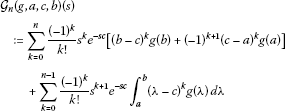(4.5)

and the remainder ${\mathcal{Z}}_{n}\left(g,a,c,b\right)\left(s\right)$ can be represented as

${\mathcal{Z}}_{n}\left(g,a,c,b\right)\left(s\right):=\frac{{\left(-1\right)}^{n+1}}{n!}{s}^{n+1}{\int }_{a}^{b}\left({\int }_{c}^{\lambda }{\left(\lambda -t\right)}^{n}{e}^{-st}\phantom{\rule{0.2em}{0ex}}dt\right)\phantom{\rule{0.2em}{0ex}}dg\left(\lambda \right).$
(4.6)

Here, $s\in \mathbb{C}$ and $c\in \left[a,b\right]$.

Since g is of bounded variation on $\left[a,b\right]$ and the derivative ${f}_{s}^{\left(n\right)}$ is Lipschitzian with the constant

${L}_{n}:={\parallel {f}_{s}^{\left(n+1\right)}\parallel }_{\left[a,b\right],\mathrm{\infty }}=|s{|}^{n+1}{\beta }_{\left[a,b\right]}\left(s\right)$

then by Theorem 2 we have the bound(4.7)

for any $s\in \mathbb{C}$ and $c\in \left[a,b\right]$.

As above, the best approximation we can get from (4.4) is for $c=\frac{a+b}{2}$, namely, we have the representation

$\left({\mathcal{L}}_{\left[a,b\right]}g\right)\left(s\right){=}_{M}{\mathcal{G}}_{n}\left(g,a,b\right)\left(s\right){+}_{M}{\mathcal{Z}}_{n}\left(g,a,b\right)\left(s\right),$
(4.8)

where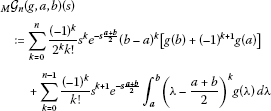(4.9)

and the remainder ${}_{M}\mathcal{Z}_{n}\left(g,a,b\right)\left(s\right)$ can be represented as

${}_{M}\mathcal{Z}_{n}\left(g,a,b\right)\left(s\right):=\frac{{\left(-1\right)}^{n+1}}{n!}{s}^{n+1}{\int }_{a}^{b}\left({\int }_{\frac{a+b}{2}}^{\lambda }{\left(\lambda -t\right)}^{n}{e}^{-st}\phantom{\rule{0.2em}{0ex}}dt\right)\phantom{\rule{0.2em}{0ex}}dg\left(\lambda \right).$
(4.10)

The error ${}_{M}\mathcal{Z}_{n}\left(g,a,b\right)\left(s\right)$ satisfies the bound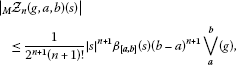(4.11)

for any $s\in \mathbb{C}$.

Now, if we restrict the function g to belong to the class of Lipschitzian functions with the constant $K>0$ on the interval $\left[a,b\right]$, then the error in the representation (4.4) will satisfy the bound

$|{\mathcal{Z}}_{n}\left(g,a,c,b\right)\left(s\right)|\le \frac{1}{\left(n+2\right)!}K|s{|}^{n+1}{\beta }_{\left[a,b\right]}\left(s\right)\left[{\left(b-c\right)}^{n+2}+{\left(c-a\right)}^{n+2}\right]$

for any $s\in \mathbb{C}$ and $c\in \left[a,b\right]$.

Finally, the error ${}_{M}\mathcal{Z}_{n}\left(g,a,b\right)\left(s\right)$ from the representation (4.8) satisfies the inequality

${|}_{M}{\mathcal{Z}}_{n}\left(g,a,b\right)\left(s\right)|\le \frac{1}{{2}^{n+1}\left(n+2\right)!}K|s{|}^{n+1}{\beta }_{\left[a,b\right]}\left(s\right){\left(b-a\right)}^{n+2}$

for any $s\in \mathbb{C}$.

1. 2.

We consider now the finite Fourier-Stieltjes sine and cosine transforms defined by

$\left({\mathcal{F}}_{s,\left[a,b\right]}g\right)\left(u\right):={\int }_{a}^{b}sin\left(ut\right)\phantom{\rule{0.2em}{0ex}}dg\left(t\right),\phantom{\rule{2em}{0ex}}\left({\mathcal{F}}_{c,\left[a,b\right]}g\right)\left(u\right):={\int }_{a}^{b}cos\left(ut\right)\phantom{\rule{0.2em}{0ex}}dg\left(t\right),$
(4.12)

where a, b are real numbers with $a, u is a real number and $g:\left[a,b\right]\to \mathbb{C}$ is a function of bounded variation.

Since the functions ${f}_{s;u},{f}_{c;u}:\left[a,b\right]\to \mathbb{R}$, ${f}_{s;u}\left(t\right):=sin\left(ut\right)$, ${f}_{c;u}\left(t\right):=cos\left(ut\right)$ are continuous for any $u\in \mathbb{R}$, the transforms (4.12) are well defined for any $u\in \mathbb{R}$.

Utilizing the well-known formulae for the n th derivatives of sine and cosine functions, namely,

and

then we have

${f}_{s;u}^{\left(k\right)}\left(t\right)={u}^{k}sin\left(ut-\frac{k\pi }{2}\right)\phantom{\rule{1em}{0ex}}\text{and}\phantom{\rule{1em}{0ex}}{f}_{c;u}^{\left(k\right)}\left(t\right)={u}^{k}cos\left(ut-\frac{k\pi }{2}\right)$

for any $u\in \mathbb{R}$ and $k\ge 0$.

We observe that, in general, we have the bounds

${\parallel {f}_{s;u}^{\left(n+1\right)}\parallel }_{\left[a,b\right],\mathrm{\infty }}=\underset{t\in \left[a,b\right]}{sup}|{u}^{n+1}sin\left(ut-\frac{\left(n+1\right)\pi }{2}\right)|\le |u{|}^{n+1}$

and

${\parallel {f}_{c;u}^{\left(n+1\right)}\parallel }_{\left[a,b\right],\mathrm{\infty }}=\underset{t\in \left[a,b\right]}{sup}|{u}^{n+1}cos\left(ut-\frac{\left(n+1\right)\pi }{2}\right)|\le |u{|}^{n+1}$

for any $u\in \mathbb{R}$, the closed interval $\left[a,b\right]$ and $n\ge 0$.

On utilizing Theorem 1, we have the representation

$\left({\mathcal{F}}_{s,\left[a,b\right]}g\right)\left(u\right)={\mathcal{K}}_{s,n}\left(g,a,c,b\right)\left(u\right)+{\mathcal{W}}_{s,n}\left(g,a,c,b\right)\left(u\right),$
(4.13)

where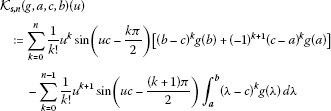(4.14)

and the remainder ${\mathcal{W}}_{s,n}\left(g,a,c,b\right)\left(u\right)$ can be represented as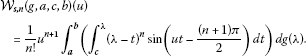(4.15)

Since g is of bounded variation on $\left[a,b\right]$ and the derivative ${f}_{s}^{\left(n\right)}$ is Lipschitzian with the constant

${L}_{n}:={\parallel {f}_{s}^{\left(n+1\right)}\parallel }_{\left[a,b\right],\mathrm{\infty }}\le |u{|}^{n+1}$

then by Theorem 2 we have the bound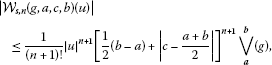(4.16)

for any $u\in \mathbb{R}$ and $c\in \left[a,b\right]$.

As above, the best approximation we can get from (4.4) is for $c=\frac{a+b}{2}$, namely, we have the representation

$\left({\mathcal{F}}_{s,\left[a,b\right]}g\right)\left(u\right){=}_{M}{\mathcal{K}}_{s,n}\left(g,a,b\right)\left(u\right){+}_{M}{\mathcal{W}}_{s,n}\left(g,a,b\right)\left(u\right),$
(4.17)

where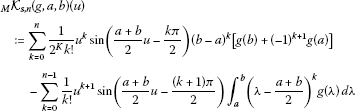(4.18)

and the remainder ${}_{M}\mathcal{W}_{s,n}\left(g,a,b\right)\left(u\right)$ can be represented as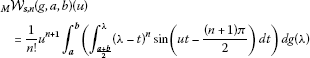(4.19)

for any $u\in \mathbb{R}$.

Here, the error satisfies the bound

${|}_{M}{\mathcal{W}}_{s,n}\left(g,a,b\right)\left(u\right)|\le \frac{1}{{2}^{n+1}\left(n+1\right)!}|u{|}^{n+1}{\left(b-a\right)}^{n+1}\underset{a}{\overset{b}{\bigvee }}\left(g\right)$
(4.20)

for any $u\in \mathbb{R}$.

Now, if we restrict the function g to belong to the class of Lipschitzian functions with the constant $K>0$ on the interval $\left[a,b\right]$, then the error in the representation (4.17) will satisfy the bound:

$|{\mathcal{W}}_{s,n}\left(g,a,c,b\right)\left(u\right)|\le \frac{1}{\left(n+2\right)!}K|u{|}^{n+1}\left[{\left(b-c\right)}^{n+2}+{\left(c-a\right)}^{n+2}\right],$
(4.21)

for any $u\in \mathbb{R}$ and $c\in \left[a,b\right]$.

Finally, the error from the representation (4.17) satisfies the inequality

${|}_{M}{\mathcal{W}}_{s,n}\left(g,a,b\right)\left(u\right)|\le \frac{1}{{2}^{n+1}\left(n+2\right)!}K|u{|}^{n+1}{\left(b-a\right)}^{n+2}$
(4.22)

for any $u\in \mathbb{R}$.

Similar results may be stated for the finite Fourier-Stieltjes cosine transform, however the details are left to the interested reader.

## References

1. Tortorella M: Closed Newton-Cotes quadrature rules for Stieltjes integrals and numerical convolution of life distributions. SIAM J. Sci. Stat. Comput. 1990, 11(4):732–748. 10.1137/0911043

2. Dubuc S, Todor F: La règle du trapèze pour l’intégrale de Riemann-Stieltjes. I. Ann. Sci. Math. Qué. 1984, 8(2):135–140. (French) [The trapezoid formula for the Riemann-Stieltjes integral. I].

3. Dubuc S, Todor F: La règle du trapèze pour l’intégrale de Riemann-Stieltjes. II. Ann. Sci. Math. Qué. 1984, 8(2):141–153. (French) [The trapezoid formula for the Riemann-Stieltjes integral. II].

4. Dubuc S, Todor F: La règle optimale du trapèze pour l’intégrale de Riemann-Stieltjes d’une fonction donnée. C. R. Math. Rep. Acad. Sci. Canada 1987, 9(5):213–218. (French) [The optimal trapezoidal rule for the Riemann-Stieltjes integral of a given function].

5. Diethelm K: A note on the midpoint rectangle formula for Riemann-Stieltjes integrals. J. Stat. Comput. Simul. 2004, 74(12):920–922.

6. Liu Z: Refinement of an inequality of Grüss type for Riemann-Stieltjes integral. Soochow J. Math. 2004, 30(4):483–489.

7. Mercer PR: Hadamard’s inequality and trapezoid rules for the Riemann-Stieltjes integral. J. Math. Anal. Appl. 2008, 344(2):921–926. 10.1016/j.jmaa.2008.03.026

8. Munteanu M: Quadrature formulas for the generalized Riemann-Stieltjes integral. Bull. Braz. Math. Soc. 2007, 38(1):39–50. 10.1007/s00574-007-0034-5

9. Mozyrska D, Pawluszewicz E, Torres DFM: The Riemann-Stieltjes integral on time scales. Aust. J. Math. Anal. Appl. 2010., 7(1): Article ID 10

10. Barnett NS, Cerone P, Dragomir SS: Majorisation inequalities for Stieltjes integrals. Appl. Math. Lett. 2009, 22: 416–421. 10.1016/j.aml.2008.06.009

11. Barnett NS, Cheung W-S, Dragomir SS, Sofo A: Ostrowski and trapezoid type inequalities for the Stieltjes integral with Lipschitzian integrands or integrators. Comput. Math. Appl. 2009, 57: 195–201. 10.1016/j.camwa.2007.07.021

12. Barnett NS, Dragomir SS: The Beesack-Darst-Pollard inequalities and approximations of the Riemann-Stieltjes integral. Appl. Math. Lett. 2009, 22: 58–63. 10.1016/j.aml.2008.02.005

13. Cerone P, Cheung W-S, Dragomir SS: On Ostrowski type inequalities for Stieltjes integrals with absolutely continuous integrands and integrators of bounded variation. Comput. Math. Appl. 2007, 54: 183–191. 10.1016/j.camwa.2006.12.023

14. Cerone P, Dragomir SS: Bounding the Čebyšev functional for the Riemann Stieltjes integral via a Beesack inequality and applications. Comput. Math. Appl. 2009, 58: 1247–1252. 10.1016/j.camwa.2009.07.029

15. Cerone P, Dragomir SS: Approximating the Riemann Stieltjes integral via some moments of the integrand. Math. Comput. Model. 2009, 49: 242–248. 10.1016/j.mcm.2008.02.011

16. Dragomir SS: Approximating the Riemann Stieltjes integral in terms of generalised trapezoidal rules. Nonlinear Anal. 2009, 71: e62-e72. 10.1016/j.na.2008.10.004

17. Dragomir SS: Inequalities for Stieltjes integrals with convex integrators and applications. Appl. Math. Lett. 2007, 20: 123–130. 10.1016/j.aml.2006.02.027

18. Dragomir SS: On the Ostrowski’s inequality for Riemann-Stieltjes integral. Korean J. Comput. Appl. Math. 2000, 7: 477–485.

19. Mitrinović DS, Pečarić JE, Fink AM: Inequalities Involving Functions and Their Integrals and Derivatives. Kluwer Academic, Dordrecht; 1991.

20. Dragomir SS, Rassias TM (Eds): Ostrowski Type Inequalities and Applications in Numerical Integration. Kluwer Academic, Dordrecht; 2002.

21. Anastassiou AG: Univariate Ostrowski inequalities, revisited. Monatshefte Math. 2002, 135(3):175–189. 10.1007/s006050200015

22. Anastassiou AG: Ostrowski type inequalities. Proc. Am. Math. Soc. 1995, 123(12):3775–3781. 10.1090/S0002-9939-1995-1283537-3

23. Aglić-Aljinović A, Pečarić J: On some Ostrowski type inequalities via Montgomery identity and Taylor’s formula. Tamkang J. Math. 2005, 36(3):199–218.

24. Aglić-Aljinović A, Pečarić J, Vukelić A: On some Ostrowski type inequalities via Montgomery identity and Taylor’s formula II. Tamkang J. Math. 2005, 36(4):279–301.

25. Bertero M, Grünbaum FA: Commuting differential operators for the finite Laplace transform. Inverse Probl. 1985, 1(3):181–192. 10.1088/0266-5611/1/3/004

26. Bertero M, Grünbaum FA, Rebolia L: Spectral properties of a differential operator related to the inversion of the finite Laplace transform. Inverse Probl. 1986, 2(2):131–139. 10.1088/0266-5611/2/2/006

27. Cheung W-S, Dragomir SS: Two Ostrowski type inequalities for the Stieltjes integral of monotonic functions. Bull. Aust. Math. Soc. 2007, 75(2):299–311. 10.1017/S0004972700039228

28. Cerone P: Approximate multidimensional integration through dimension reduction via the Ostrowski functional. Nonlinear Funct. Anal. Appl. 2003, 8(3):313–333.

29. Cerone P, Dragomir SS: On some inequalities arising from Montgomery’s identity. J. Comput. Anal. Appl. 2003, 5(4):341–367.

30. Kumar P: The Ostrowski type moment integral inequalities and moment-bounds for continuous random variables. Comput. Math. Appl. 2005, 49(11–12):1929–1940. 10.1016/j.camwa.2003.11.002

31. Pachpatte BG: A note on Ostrowski like inequalities. J. Inequal. Pure Appl. Math. 2005., 6(4): Article ID 114

32. Sofo A: Integral inequalities for N -times differentiable mappings. In Ostrowski Type Inequalities and Applications in Numerical Integration. Kluwer Academic, Dordrecht; 2002:65–139.

33. Ujević N: Sharp inequalities of Simpson type and Ostrowski type. Comput. Math. Appl. 2004, 48(1–2):145–151. 10.1016/j.camwa.2003.09.026

34. Dragomir SS: Ostrowski’s inequality for montonous mappings and applications. J. KSIAM 1999, 3(1):127–135.

35. Dragomir SS: Some inequalities for Riemann-Stieltjes integral and applications. In Optimization and Related Topics. Edited by: Rubinov A, Glover B. Kluwer Academic, Dordrecht; 2001:197–235.

36. Dragomir, SS: Accurate approximations of the Riemann-Stieltjes integral with $\left(l,L\right)$-Lipschitzian integrators. In: Simos, TH, et al. (eds.) AIP Conf. Proc. 939, Numerical Anal. & Appl. Math., pp. 686–690. Preprint RGMIA Res. Rep. Coll. 10(3), Article ID 5 (2007). Online http://rgmia.vu.edu.au/v10n3.html

37. Dragomir SS: Accurate approximations for the Riemann-Stieltjes integral via theory of inequalities. J. Math. Inequal. 2009, 3(4):663–681.

38. Dragomir, SS: Approximating the Riemann-Stieltjes integral by a trapezoidal quadrature rule with applications. Math. Comput. Model. (in Press). Corrected Proof, Available online 18 February 2011

39. Dragomir SS, Buşe C, Boldea MV, Brăescu L: A generalisation of the trapezoidal rule for the Riemann-Stieltjes integral and applications. Nonlinear Anal. Forum 2001, 6(2):337–351.

40. Dragomir SS, Cerone P, Roumeliotis J, Wang S: A weighted version of Ostrowski inequality for mappings of Hölder type and applications in numerical analysis. Bull. Math. Soc. Sci. Math. Roum. 1999, 42(90)(4):301–314.

41. Dragomir SS, Fedotov I: An inequality of Grüss type for the Riemann-Stieltjes integral and applications for special means. Tamkang J. Math. 1998, 29(4):287–292.

42. Dragomir SS, Fedotov I: A Grüss type inequality for mappings of bounded variation and applications to numerical analysis. Nonlinear Funct. Anal. Appl. 2001, 6(3):425–433.

43. Pachpatte BG: A note on a trapezoid type integral inequality. Bull. Greek Math. Soc. 2004, 49: 85–90.

44. Ujević N: Error inequalities for a generalized trapezoid rule. Appl. Math. Lett. 2006, 19(1):32–37. 10.1016/j.aml.2005.03.005

45. Wu Q, Yang S: A note to Ujević’s generalization of Ostrowski’s inequality. Appl. Math. Lett. 2005, 18(6):657–665. 10.1016/j.aml.2004.08.010

46. Dragomir SS: On the Ostrowski inequality for Riemann-Stieltjes integral${\int }_{a}^{b}f\left(t\right)\phantom{\rule{0.2em}{0ex}}du\left(t\right)$ , where f is of Hölder type and u is of bounded variation and applications. J. KSIAM 2001, 5(1):35–45.

47. Cerone P, Dragomir SS, et al.: New bounds for the three-point rule involving the Riemann-Stieltjes integral. In Advances in Statistics, Combinatorics and Related Areas. Edited by: Gulati C. World Scientific, Singapore; 2002:53–62.

49. Miletic J: A finite Laplace transform method for the solution of a mixed boundary value problem in the theory of elasticity. J. M éc. Appl. 1980, 4(4):407–419.

50. Rutily B, Chevallier L: The finite Laplace transform for solving a weakly singular integral equation occurring in transfer theory. J. Integral Equ. Appl. 2004, 16(4):389–409. 10.1216/jiea/1181075298

51. Valbuena M, Galue L, Ali I: Some properties of the finite Laplace transform. In Transform Methods & Special Functions, Varna ’96. Bulgarian Acad. Sci., Sofia; 1998:517–522.

52. Watanabe K, Ito M: A necessary condition for spectral controllability of delay systems on the basis of finite Laplace transforms. Int. J. Control 1984, 39(2):363–374. 10.1080/00207178408933171

## Acknowledgements

Dedicated to Professor Hari M Srivastava.

The authors would like to thank the anonymous referees for the valuable suggestions that have been incorporated in the final version of the paper.

## Author information

Authors

### Corresponding author

Correspondence to SS Dragomir.

### Competing interests

The authors declare that they have no competing interests.

### Authors’ contributions

The authors SSD and SA have contributed equally in all stages of writing the paper.

## Rights and permissions

Reprints and Permissions

Dragomir, S., Abelman, S. Approximating the Riemann-Stieltjes integral of smooth integrands and of bounded variation integrators. J Inequal Appl 2013, 154 (2013). https://doi.org/10.1186/1029-242X-2013-154

• Accepted:

• Published:

• DOI: https://doi.org/10.1186/1029-242X-2013-154

### Keywords

• Riemann-Stieltjes integral
• Taylor’s representation
• functions of bounded variation
• Lipschitzian functions
• integral transforms
• finite Laplace-Stieltjes transform
• finite Fourier-Stieltjes sine and cosine transforms